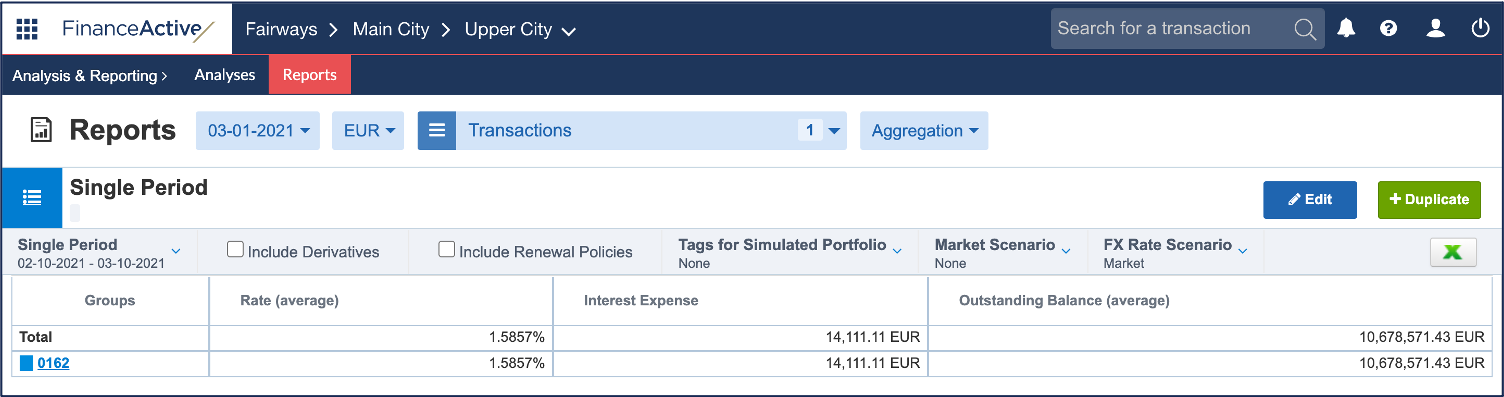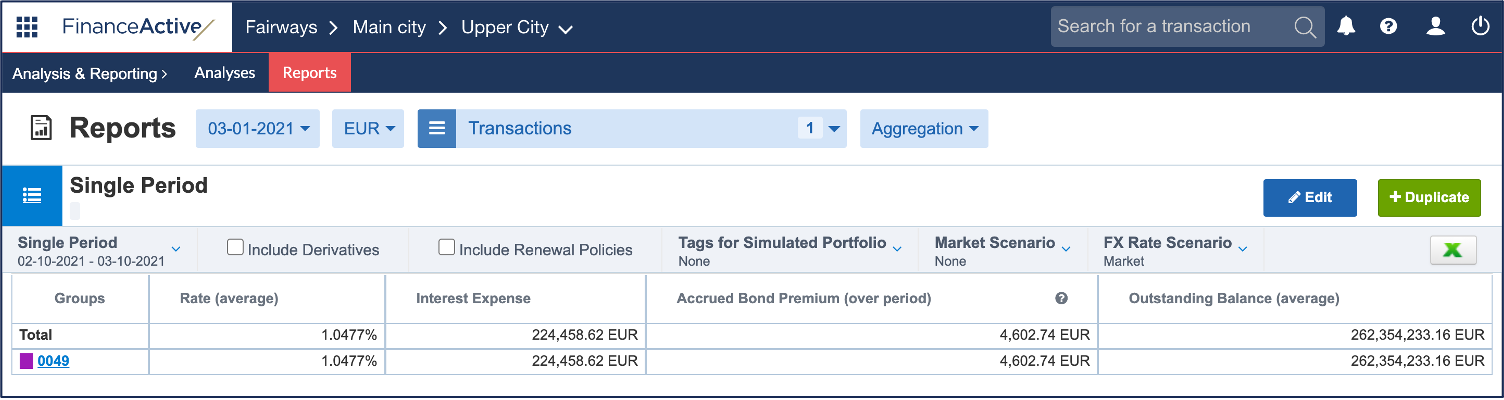# Rate (average)

In Fairways Debt, the average rate is the funding rate for the analysis period without taking fees into account. This rate is converted into a uniform interest rate method: 30/360 US.

## Periodic / Single Period Reports

Average rate:

Interest Expense / ( Average Outstanding Balance * Count of days in the report period assuming there are 30 days in a month / 360)

For example, the average rate of 0162 is 1,5857%:

Interest Expense / ( Average Outstanding Balance * Count of days in the report period assuming there are 30 days in a month / 360)

= 14 111,11 / (10 678 571,43 * 30 / 360)

= 1,5857While there are actually only 28 days from 10 February to 10 March 2021, using the 30/360 US convention, we assume all months last 30 days.

The computation of bonds includes the accrued bond premium over the period:

(Interest Expense + Accrued Bond Premium over Period) / ( Average Outstanding Balance * Count of days in the report period assuming there are 30 days in a month / 360)

For example, the average rate of 0049 is 1,0477%:

(Interest Expense + Accrued Bond Premium over Period) / ( Average Outstanding Balance * Count of days in the report period assuming there are 30 days in a month / 360)

= (224 458,62 + 4 602,74) / (262 354 233,16 * 30 / 360)

= 1,0477While there are actually only 28 days from 10 February to 10 March 2021, using the 30/360 US convention, we assume all months last 30 days.